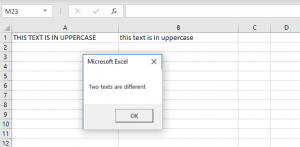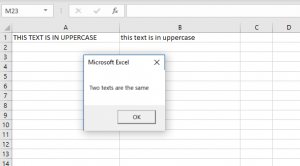# Prevent VBA Case Sensitive – Option Compare Text

While working in VBA, you often need to compare strings. By default, VBA is case sensitive, so if you compare two same texts and one of them is in upper case and other in lower case, VBA will consider them as different texts. In this tutorial, you will see how to prevent VBA from being case sensitive.

## Making VBA Case Insensitive

In order to make VBA case-insensitive, you need to put Option Compare Text at the beginning of the module. First, we will look at the standard VBA behavior without this option set. This is the code:

``````If Sheet1.Range("A1").Value = Sheet1.Range("B1").Value Then
MsgBox "Two texts are the same"
Else
MsgBox "Two texts are different"
End If``````

In the example, we want to compare the strings from the A1 and B1. If the strings are equal, we will return the message box with the message “Two texts are the same”. If they are not equal, we will return the message “Two texts are different.Image 1. Comparing the strings without the Option Compare Text

As you can see in the picture, both texts are the same, but the first one is in upper case, while the second is in lower case. Because of that, VBA considers them different and returned this message.

``````Option Compare Text

Public Sub CaseSensitiveTest()

If Sheet1.Range("A1").Value = Sheet1.Range("B1").Value Then
MsgBox "Two texts are the same"
Else
MsgBox "Two texts are different"
End If

End Sub
``````

Now we will add Option Compare Text at the beginning of the module and see the difference. Here is the code. We run the code on the same example:Image 2. Comparing the strings with the Option Compare Text

Now, when the Option Compare Text is set, the VBA becomes case insensitive and considers these two texts the same.

## Comparing Text

If you don’t declare Option Compare Text, you can convert string cases in order to make case-insensitive comparisons. This is possible by UCase, LCase or StrConv function. You can find out more about this here: VBA Upper, Lower, and Proper Case – Case Functions

Stop searching for VBA code online. Learn more about AutoMacro - A VBA Code Builder that allows beginners to code procedures from scratch with minimal coding knowledge and with many time-saving features for all users!